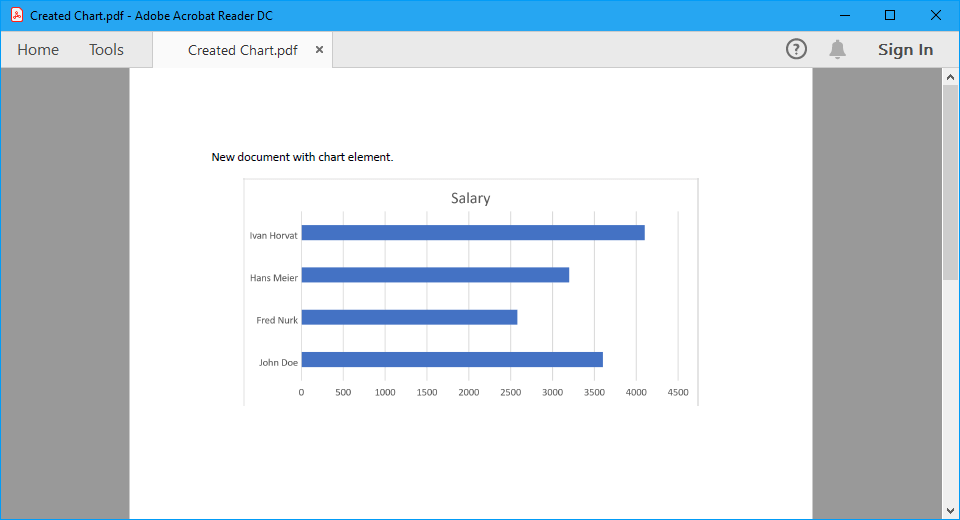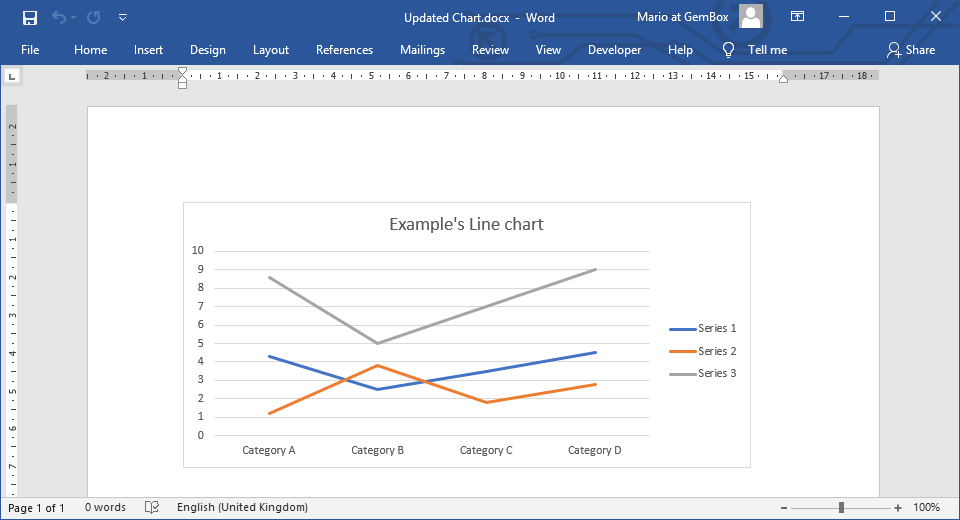# Word Charts in C# and VB.NET

`Chart` elements are drawings used for graphical data representation. GemBox.Document provides support for working with charts programmatically with the help of the GemBox.Spreadsheet component.

• With With GemBox.Spreadsheet, GemBox.Document can create and update charts in Word documents. It can also export documents with graphs to PDF, XPS, or image formats.
• Without GemBox.Spreadsheet, GemBox.Document is only able to preserve charts in Word documents.

## Create chart

To enable GemBox.Document's support and work with `Chart` objects, you'll need to add a reference to GemBox.Spreadsheet library and call its `SpreadsheetInfo.SetLicense` method.

Note, if you don't have a GemBox.Spreadsheet license you can use its Free mode.

You can choose to create charts from the following types:

• Area
• Bar
• Column
• Combo
• Line
• Pie
• Scatter

The following example shows how to create a document with a bar chart using C# and VB.NET.Screenshot of PDF document with chart
``````using GemBox.Document;

class Program
{
static void Main()
{
// If using Professional version, put your GemBox.Document serial key below.

// If using Professional version, put your GemBox.Spreadsheet serial key below.

var document = new DocumentModel();

// Create Word chart and add it to document.
var chart = new Chart(document, GemBox.Document.ChartType.Bar,
new FloatingLayout(
new HorizontalPosition(HorizontalPositionType.Center, HorizontalPositionAnchor.Margin),
new VerticalPosition(VerticalPositionType.Top, VerticalPositionAnchor.Paragraph),
new Size(14, 7, GemBox.Document.LengthUnit.Centimeter)));

new Section(document,
new Paragraph(document, "New document with chart element."),
new Paragraph(document, chart)));

// Get underlying Excel chart.
ExcelChart excelChart = (ExcelChart)chart.ExcelChart;
ExcelWorksheet worksheet = excelChart.Worksheet;

// Add data for Excel chart.
worksheet.Cells["A1"].Value = "Name";
worksheet.Cells["A2"].Value = "John Doe";
worksheet.Cells["A3"].Value = "Fred Nurk";
worksheet.Cells["A4"].Value = "Hans Meier";
worksheet.Cells["A5"].Value = "Ivan Horvat";

worksheet.Cells["B1"].Value = "Salary";
worksheet.Cells["B2"].Value = 3600;
worksheet.Cells["B3"].Value = 2580;
worksheet.Cells["B4"].Value = 3200;
worksheet.Cells["B5"].Value = 4100;

// Select data.
excelChart.SelectData(worksheet.Cells.GetSubrange("A1:B5"), true);

document.Save("Created Chart.%OutputFileType%");
}
}
``````
``````Imports GemBox.Document

Module Program

Sub Main()

' If using Professional version, put your GemBox.Document serial key below.

' If using Professional version, put your GemBox.Spreadsheet serial key below.

Dim document As New DocumentModel()

' Create Word chart and add it to document.
Dim chart As New Chart(document, GemBox.Document.ChartType.Bar,
New FloatingLayout(
New HorizontalPosition(HorizontalPositionType.Center, HorizontalPositionAnchor.Margin),
New VerticalPosition(VerticalPositionType.Top, VerticalPositionAnchor.Paragraph),
New Size(14, 7, GemBox.Document.LengthUnit.Centimeter)))

New Section(document,
New Paragraph(document, "New document with chart element."),
New Paragraph(document, chart)))

' Get underlying Excel chart
Dim excelChart As ExcelChart = DirectCast(chart.ExcelChart, ExcelChart)
Dim worksheet As ExcelWorksheet = excelChart.Worksheet

' Add data for Excel chart.
worksheet.Cells("A1").Value = "Name"
worksheet.Cells("A2").Value = "John Doe"
worksheet.Cells("A3").Value = "Fred Nurk"
worksheet.Cells("A4").Value = "Hans Meier"
worksheet.Cells("A5").Value = "Ivan Horvat"

worksheet.Cells("B1").Value = "Salary"
worksheet.Cells("B2").Value = 3600
worksheet.Cells("B3").Value = 2580
worksheet.Cells("B4").Value = 3200
worksheet.Cells("B5").Value = 4100

' Select data.
excelChart.SelectData(worksheet.Cells.GetSubrange("A1:B5"), True)

document.Save("Created Chart.%OutputFileType%")

End Sub
End Module``````

For more information about charts, visit the Chart Components and Chart Formatting examples.

## Update chart

The following example shows how you can update line chart data in a Word document.Screenshot of Word document with updated chart
``````using System.Linq;
using GemBox.Document;

class Program
{
static void Main()
{
// If using Professional version, put your GemBox.Document serial key below.

// If using Professional version, put your GemBox.Spreadsheet serial key below.

var document = DocumentModel.Load("%#Chart.docx%");

// Get Word chart.
var chart = (Chart)document.GetChildElements(true, ElementType.Chart).First();

// Get underlying Excel chart and cast it as LineChart.
var lineChart = (LineChart)chart.ExcelChart;

// Add new line series which has doubled values from the first series.
.Values.Cast<double>().Select(val => val * 2));

document.Save("Updated Chart.%OutputFileType%");
}
}
``````
``````Imports System.Linq
Imports GemBox.Document

Module Program

Sub Main()

' If using Professional version, put your GemBox.Document serial key below.

' If using Professional version, put your GemBox.Spreadsheet serial key below.

Dim document = DocumentModel.Load("%#Chart.docx%")

' Get Word chart.
Dim chart = DirectCast(document.GetChildElements(True, ElementType.Chart).First(), Chart)

' Get underlying Excel chart and cast it as LineChart.
Dim lineChart = DirectCast(chart.ExcelChart, LineChart)

' Add new line series which has doubled values from the first series.
lineChart.Series.Add("Series 3", lineChart.Series.First() _
.Values.Cast(Of Double)().Select(Function(val) val * 2))

document.Save("Updated Chart.%OutputFileType%")

End Sub
End Module``````

Some chart types are not supported through an API (like 3D charts) and won't be represented with `Chart` object.

You can find a list of supported chart types on GemBox.Spreadsheet's Charts help page.